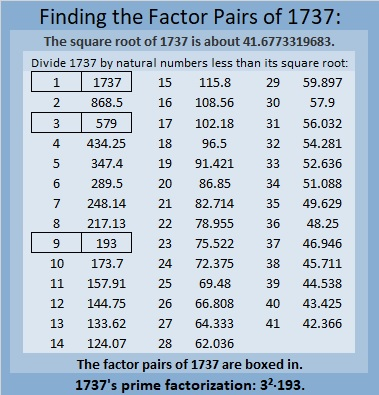# 1737 What Logic Will You Use to Solve This Puzzle?

Contents

### Today’s Puzzle:

Place each number from 1 to 12 in both the first column and the top row so that those numbers are the factors of the given clues. This is a level 6 puzzle so the logic needed to solve the puzzle will be a little more complicated. Good luck!### Factors of 1737:

1 + 7 + 3 + 7 = 18, a number divisible by nine, so 1718 is divisible by 9.

• 1737 is a composite number.
• Prime factorization: 1737 = 3 × 3 × 193, which can be written 1737 = 3² × 193.
• 1737 has at least one exponent greater than 1 in its prime factorization so √1737 can be simplified. Taking the factor pair from the factor pair table below with the largest square number factor, we get √1737 = (√9)(√193) = 3√193.
• The exponents in the prime factorization are 2 and 1. Adding one to each exponent and multiplying we get (2 + 1)(1 + 1) = 3 × 2 = 6. Therefore 1737 has exactly 6 factors.
• The factors of 1737 are outlined with their factor pair partners in the graphic below.### More About the Number 1737:

1737 is the difference of two squares in three ways:
869² – 868² = 1737,
291² – 288² = 1737, and
101² – 92² = 1737.

1737 is the sum of consecutive numbers:
868 + 869 = 1737,
578 + 579 +560 = 1737,
287 + 288 + 289 + 290 + 291 + 292 = 1737,
189 + 190 + 191 + 192 + 193 + 194 + 195 + 196 + 197 = 1737, and
88+89+90+91+92+93+94+95+96+97+98+99+100+101+102+103+104+105=1737.

That first sum uses the same numbers as the first difference of two squares. Check out these square equations!

292² – 291² + 290² – 289² + 288² – 287² = 1737.

105² – 104² + 103² – 102² + 101² – 100² + 99² – 98² + 97² – 96² + 95² – 94² + 93² – 92² + 91² – 90² + 89² – 88² = 1737.

(But since 101² – 92² = 1737, we can conclude that all the rest of the numbers added and subtracted will give us zero!)

1737 is also the sum of two squares:
36² + 21² = 1737.

1737 is the hypotenuse of a Pythagorean triple:
855-1512-1737 calculated from 36² – 21², 2(36)(21), 36² + 21².
That triple is also 9 times (95-168-193).

That Pythagorean triple means that
855² + 1512² = 1737².

From OEIS.org, we learn that when we add up these three consecutive squares,
1736² + 1737² + 1738² we get a palindrome, specifically, 9051509. Cool!

1737² = 1736² + 1734² – 1733² + 1732² – 1731² – 1729² + 1728².

This site uses Akismet to reduce spam. Learn how your comment data is processed.